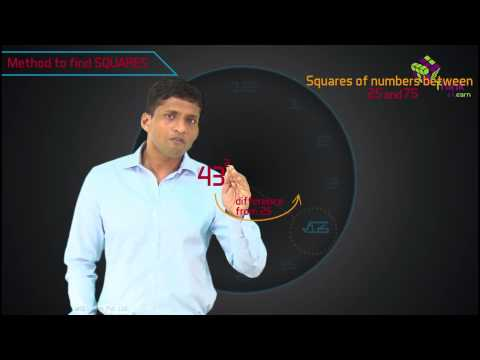# Finding The Square Root Of Decimals

Any number can be expressed as a product of prime numbers. This method of representation of a number in terms of product of prime numbers is termed as prime factorization method. It is the easiest method known for the manual calculation of square root of a number. But this method becomes tedious and tiresome when the number involved is large. In order to overcome this problem we use the long division method. The number of digits in a perfect square is very significant for calculating its square root by long division method. Consider the following method for finding the square root of a decimal number. It is explained with the help of an example for a clear understanding.

## Finding Square root of Decimals

1. Consider the number 24.01 whose square root is to be calculated. We put the bar on the integral part of the number starting from the unit’s digit. This bar is placed in pairs. Similarly for decimal part, we put the bar on the pair of numbers starting from the digit which is immediately placed after the decimal.

$$\begin{array}{l}\text{In this case, it will be}\ \overline{24}.\ \overline{01}.\end{array}$$

2. The divisor will be the maximum number whose square is less than or equal to the dividend that is the integral part of the number. In this case the divisor will be 4 and the dividend is 24. Divide and get the remainder.3. The remainder in this case is 8. Write the number which is in the next bar to the right side of the remainder. We get 801.
4. Double the value of the divisor and enter it with a blank on its right. Since 01 is the decimal part of the number, put a decimal point in the quotient.5. Now we have to select the largest digit for the unit place of the divisor (8_) such that the new number when multiplied by the new digit at unit’s place is equal to or less than the dividend (801). As we can see that 89 × 9 = 801, the new digit of the divisor is 9 and thus the divisor is 89. Divide the number and get the remainder.6. Since the remainder is 0 and no bar is left, therefore we can say that √24.01 = 4.9